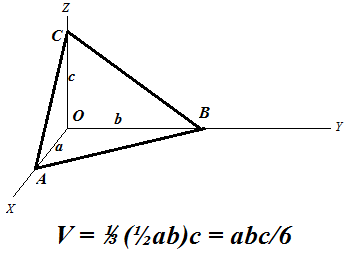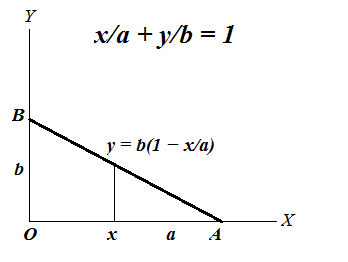## Monday, August 21, 2017

### Unizor - Double Integrals - Volume of Pyramid

Notes to a video lecture on http://www.unizor.com

Double Integrals - Pyramid

As another example of a double integral as a volume of a solid, consider a pyramid with three plains coinciding with three coordinate planes as follows:
base is a right triangle with vertex of the right angle at the origin of coordinates O and catheti OA (along the X-axis) and OB (along the Y-axis);
point C on the Z-axis is the top vertex of this pyramid.

Let the lengths of these sides be
OA = a
OB = b
OC = c
Our task is to calculate the volume of this pyramid using the double integral and techniques described in the previous lecture.We can consider this problem as the problem of determining a volume of a solid bounded on the top by a plane going through three given points AB and C and defined on the base triangle ΔOAB lying in the XY-plane.

Since we intend to integrate a function to determine a volume, let's express the plane going through points AB and C as a function f(x,y) of two arguments x and y - coordinates of a point that belong to a base triangle.
Traditional equation of this plane in three-dimensional coordinates is
x/a + y/b + z/c = 1
from which follows the functional representation
z = f(x, y) = c·(1 − x/a − y/b)

Since our base in not rectangular, we have to pick the primary argument, let it be x changing from 0 to a. The argument y has limits that depend on the value of x in order for point (x, y) to be within our triangle. For each x, therefore, argument y should change from 0 to a point on the hypotenuse of our base triangle.
Equation of this line on a plane that goes through points A on the X-axis and B on the Y-axis is
x/a + y/b = 1
Therefore, the point on a hypotenuse that corresponds to X-coordinate x is
y = b·(1 − x/a)So, for each primary coordinate x (from 0 to a) coordinate y is changing from 0 to b·(1 − x/a).

Using exactly the same logic as in the previous lecture, we can express the volume of this pyramid as a double integral of function
f(x, y) = c·(1 − x/a − y/b)
on a base triangle ΔOAB described by primary argument x changing from 0 to a and dependent argument y changing from 0 to b·(1 − x/a).

Now the formula for a volume of our pyramid would be
V = 0a [∫0b·(1−x/a) f(x,y) dy] dx
where
f(x, y) = c·(1 − x/a − y/b)

Let's perform the inner integration by y considering x constant.
0b·(1−x/a) f(x,y) dy =
0b·(1−x/a)c·(1−x/a−y/b) dy =
c·(1−x/a) 0b·(1−x/a) dy − (c/b) 0b·(1−x/a)dy =
b·c·(1−x/a)²−b·c·(1−x/a)²/2 =
b·c·(1−x/a)²/2
Now, to get the volume of our pyramid, we have to integrate this as a function of x from 0 to a:
V = 0a b·c·(1−x/a)²/2 dx
In this case, to integrate it, let's make a substitution
t = 1−x/a
Then we can express x, its differential dx and limits of integration 0 and a in terms of t:
x = a − a·t
dx = −a·dt
x=0  t=1
x=a  t=0

As a result of this substitution,
V = 10 b·c·t²/2 (−a)·dt =
(a·b·c/2)·01dt =
(a·b·c/2)·(1/3) = a·b·c/6
which corresponds to a known formula for a volume of a pyramid.